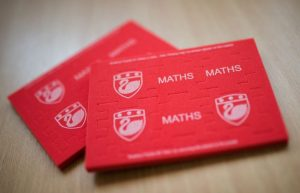## Maths at PauletWhat do students learn in Maths in Key Stage 3?

In KS3, students learn a variety of topics including Algebra, Number, Shape and Mathematical Reasoning

Students

• become fluent in the fundamentals of mathematics, including through varied and frequent practice with increasingly complex problems over time, so that students develop conceptual understanding and the ability to recall and apply knowledge rapidly and accurately
• reason mathematically by following a line of enquiry, conjecturing relationships and generalisations and developing an argument, justification or proof using mathematical language
• can solve problems by applying their mathematics to a variety of routine and non-routine problems with increasing sophistication, including breaking down problems into a series of simpler steps and persevering in seeking solutions.

The programme of study for Key Stage 3 is organised into distinct units that build on students’ Key Stage 2 knowledge.  Students are encouraged to make connections across mathematical ideas to develop fluency, mathematical reasoning and competence in solving increasingly sophisticated problems.

How do students learn in KS3 Maths?

In KS3 maths we follow the White Rose Scheme of Work. This focuses on algebra and number skills for the first year in secondary school and then moves onto shape, probability and the other elements of maths. Worksheets and textbooks are widely used as well as other resources that provide appropriate challenge and scaffold to those students that require.

What topics are covered and when in each year group? What is the sequence of teaching?

 Year 7 Year 8 Year 9 Autumn 1 Algebra Proportional Reasoning Number Autumn 2 Place Value and Proportion Respresentations Algebra Spring 1 Application of Number Algebraic Techniques Statistics Spring 2 Directed Numbers and Fractions Developing Number Fractions Summer 1 Lines and Angles Developing Geometry Angles and Pythagoras Summer 2 Reasoning with Number Reasoning with Data Graphs

How are students assessed in KS3 Maths?

Students are offered feedback in all lessons and after every assessment point.

Each half term students will receive an assessed piece of homework and a half term assessment in class. This will be used by teachers to decide if students are on track to meeting their targets.

Homework

Homework is issued on MathsWatch where all students have an account similar to their school emails. This is live all year and any feedback is given immediately to help students improve.